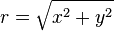# Grey-box Models

The idea of 'grey-box' models would be to give some way in which complicated sub-models could be expressed with intermediate variables, without needing to change the system's higher-level structure.

To some extent this is like explicit 'tearing' of the system of equations, as it delimits a block in which any internal variables must be solved before the higher-level system gets to see the remaining variables referenced by that block.

The current ASCEND system for dealing with 'black box' elements would be a suitable API for allowing the solver to deal with these, hence the name 'grey box': the modeller can see the equations but the top-level solver can not, and must deal with the equations as though they were a black box.

## Example

Perhaps we wish to solve a problem involving the radius of a circle$r= \sqrt{x^2+y^2}$

We could just write

MODEL circle;
x,y,r IS_A solver_var;

r_eq: r = sqrt(x^2 + y^2);

MODEL;


but what if that r_eq were more complicated. Perhaps then we would like to write it separately and include some intermediate values in the calculation:

MODEL radius;
x,y,r IS_A solver_var;

x2,y2 IS_A solver_var;
x2 = x^2;

y2 = y^2;
r = x2 + y2;